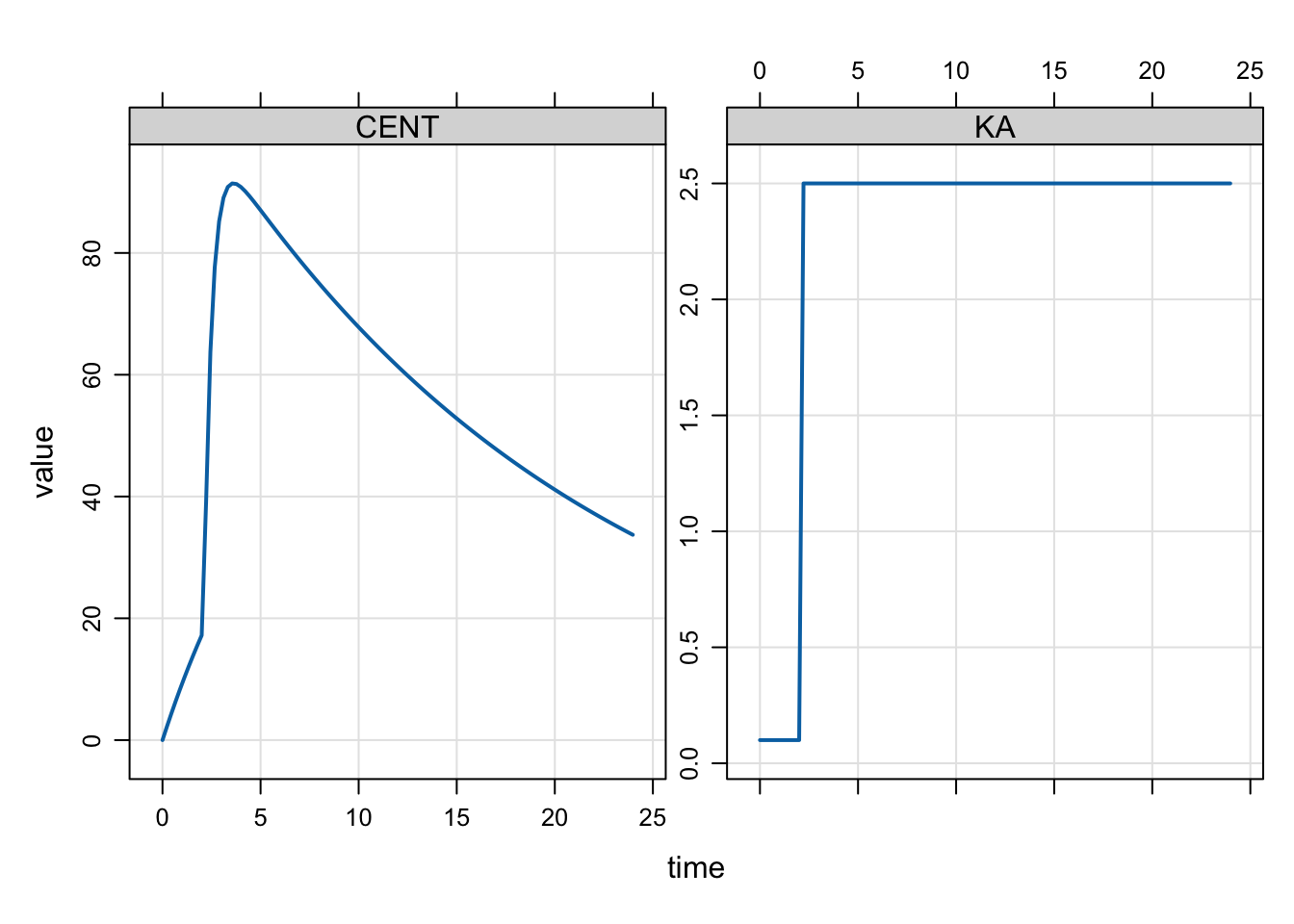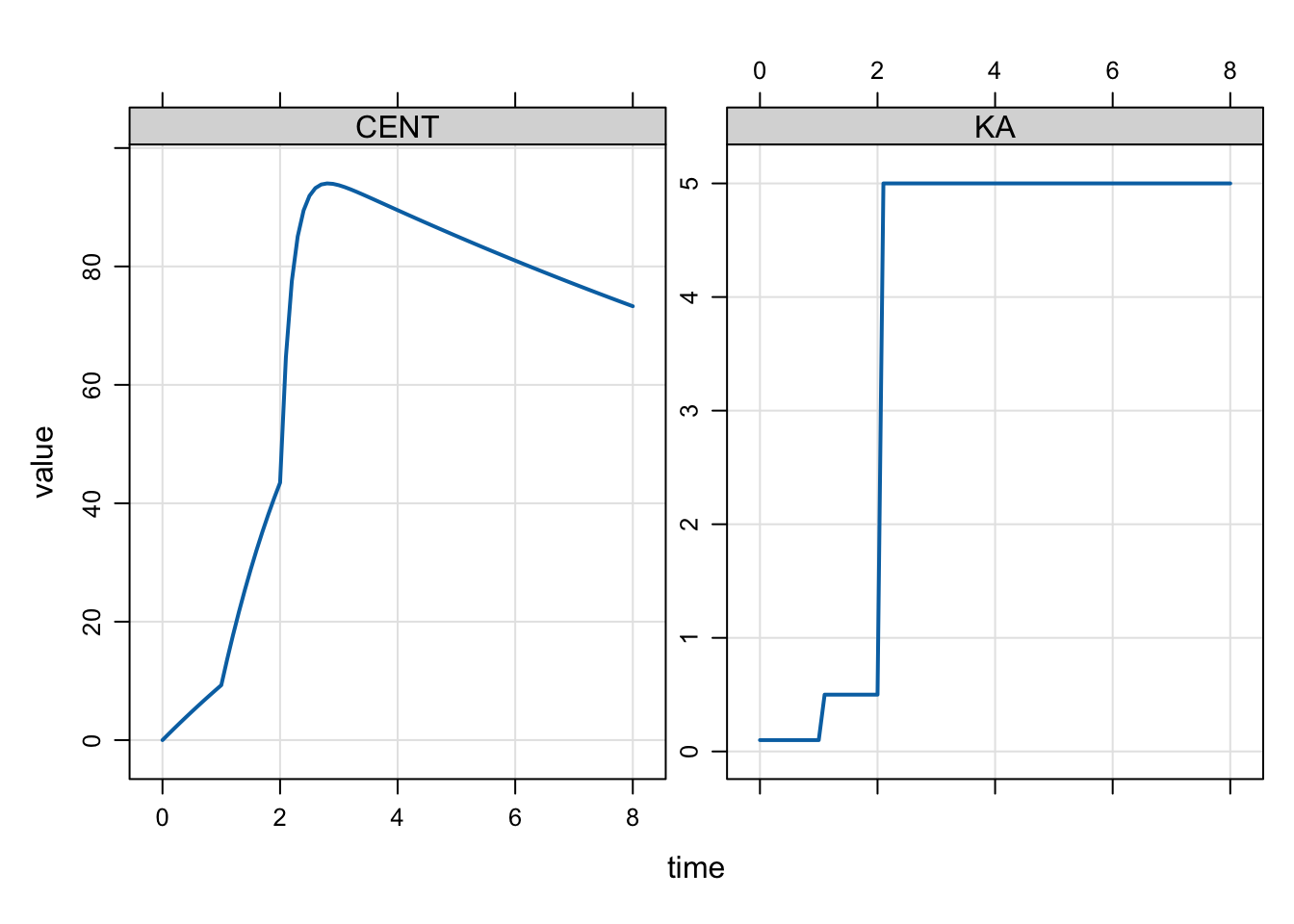# mtime

This is just a post on a simplified implementation of modeled events in mrgsolve. There is also another, more complete blog post on this topic published previously .

modeled events
mtime
Author

Kyle Baron

Published

January 1, 2018

This is just a short post on a simplified implementation of modeled events in mrgsolve.

I previously posted here about modeling interventions in mrgsolve. All that still works fine. But I found myself wanting a more straightforward implementation of the mtime functionality you get in NONMEM.

# 1 Example with single mtime

For example, we might wish a rate constant to change at some time that is not found in a record in the input data set. We can make this happen from the model code itself. In addition to using `mevent` you can now use `mtime` to make this happen. `mtime` is just a simpler subset of the `mevent` functionality.

Let’s make KA change at 2.1 hours (`change_t` parameter in the example below).

``````[ param ]
CL = 1, V = 20, KA1 = 0.1, KA2 = 2.5, change_t = 2.1

[ pkmodel ]
cmt = "GUT,CENT", depot = TRUE

[ main ]
double mt = self.mtime(change_t);

capture KA = KA1;

if(TIME > mt) KA = KA2;

if(TIME == change_t) {
mrg::report("wait a minute ... time is 2.1?");
}``````

Again, the main motivation for this is just convenience and economy of code: we register the event time and get that time returned into a variable that we can reference later on, checking if we are past that time or not.

``````library(mrgsolve)

options(mrgsolve.soloc = "build")

mod %>% ev(amt=100) %>% mrgsim(delta = 0.222) %>% plot(CENT+KA~time)``````You won’t see the message that we actually stumbled on `2.1` hours in the simulation even though it was not in the lineup when the simulation started.

# 2 Example with several mtimes

We could keep track of several mtimes like this

``````[ set ] end=8, delta=0.1

[ param ]
CL = 1, V = 20, KA1 = 0.1, KA2 = 0.5, KA3 = 5, change_t = 1

[ pkmodel ]
cmt = "GUT,CENT", depot = TRUE

[ main ]
double mt = self.mtime(change_t);

double mt2 = self.mtime(change_t + 1);

capture KA = KA1;

if(TIME > mt) KA = KA2;

if(TIME > mt2) KA = KA3;``````
``````mod <- mread_cache("mtime_model_2.txt")

mod %>% ev(amt=100) %>% mrgsim() %>% plot(CENT + KA ~ time)``````